## 什么是Online Learning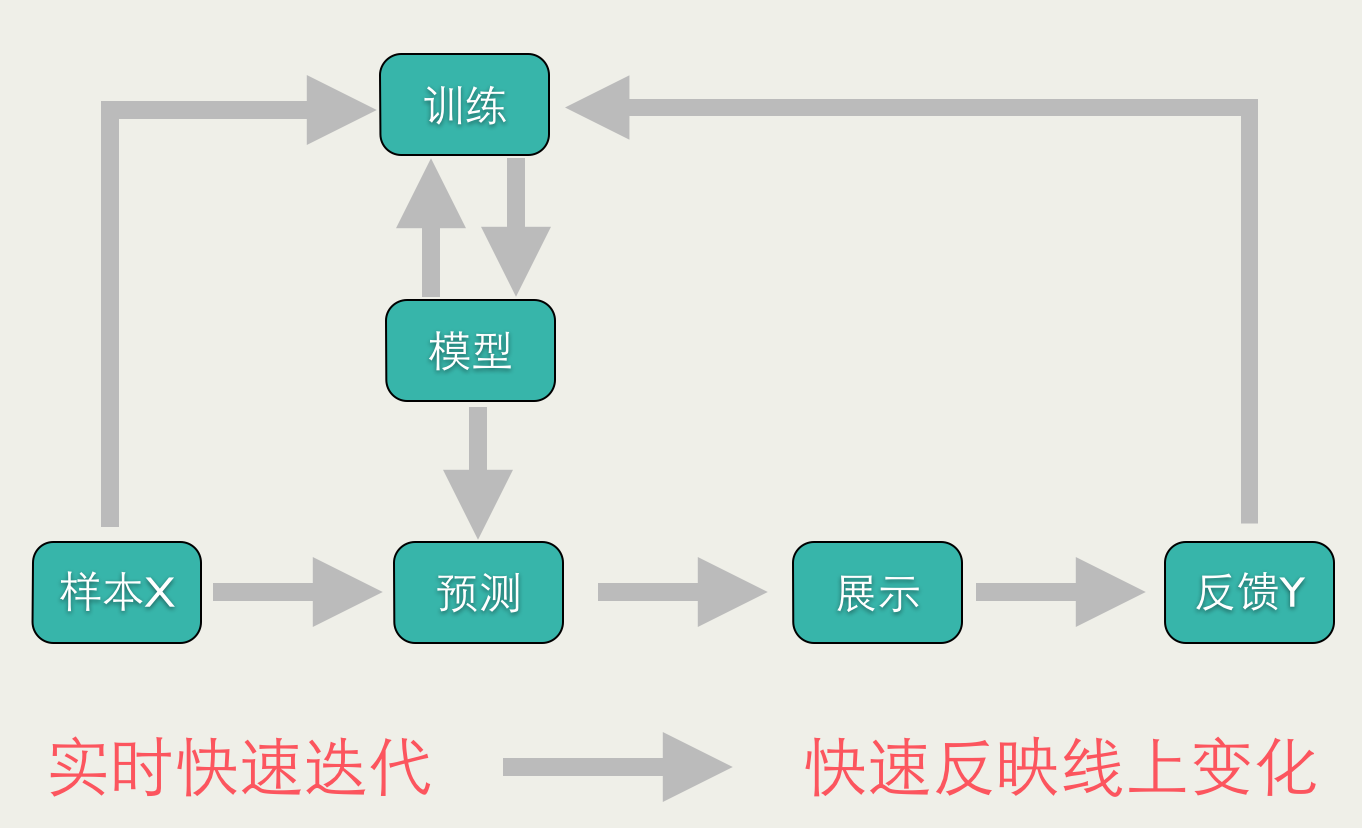Online Learning有点像自动控制系统，但又不尽相同，二者的区别是：Online Learning的优化目标是整体的损失函数最小化，而自动控制系统要求最终结果与期望值的偏差最小。

###### Online Learning的优化目标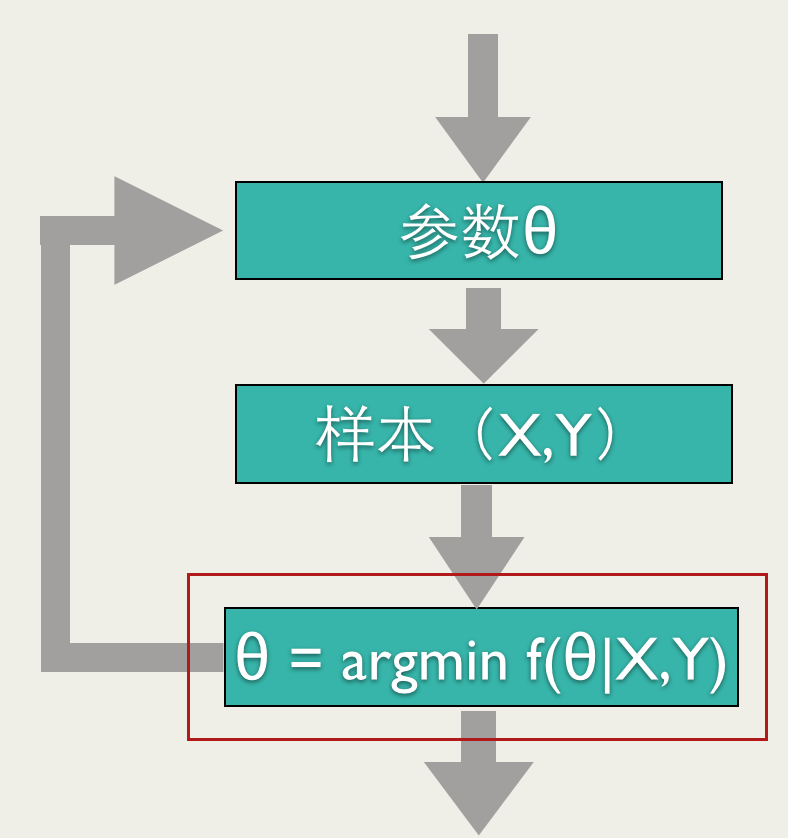## 怎样实现Online Learning

###### Bayesian Online Learning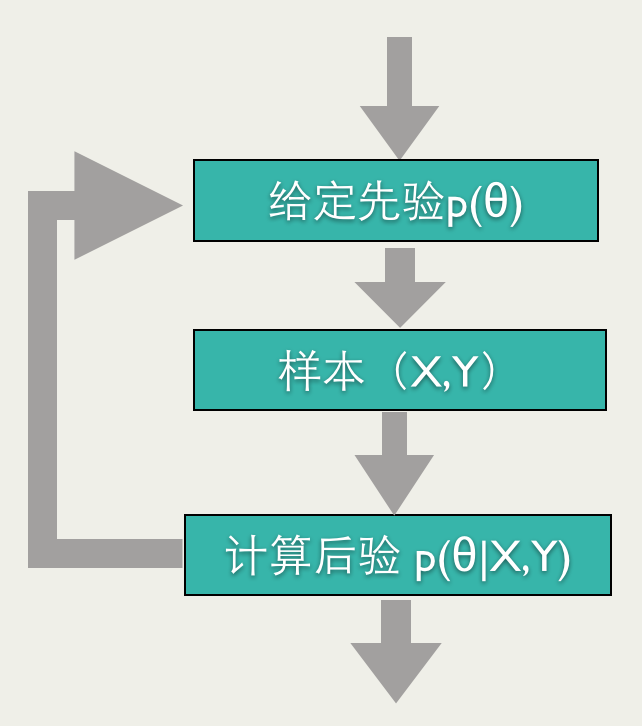Bayesian Online Learning最常见的应用就是BPR（Bayesian Probit Regression）。

###### BPR

$$p \left( y \right) = N \left( y \mid A\mu_X +b, \Sigma_y + A \Sigma_x A^{T} \right)$$

$$Y$$是一维变量，是$$w$$与特征向量$$x$$的内积，加入方差为$$\beta^{2}$$的扰动：

$$p\left( y \mid w\right) = N(y \mid x^Tw, \beta^2)$$ 根据上面的式子可以得出： $$p\left( y \mid w\right) = N(y \mid x^T\mu, x^T\Sigma x +\beta^2)$$ 由于我们只能观测到$$Y$$，是大于0，还是小于0，即$$Y_{i} \in \{-1，1\}$$,$$Y_{i} = -1$$，代表观测值小于0，$$Y_{i} = 1$$代表观测值大于0。

$$\tilde \sigma_{d} = \sigma_{d} \cdot \left[ 1 - x_{i,d} \cdot \frac{\sigma_{d}^{2}}{x^T\Sigma x +\beta^2} \omega\left(Y_{i} \cdot \frac{x^T\mu}{\sqrt{x^T\Sigma x +\beta^2}} \right) \right]$$

Online Bayesian Probit Regression 训练流程如下： >初始化 $$\mu_{1}$$,$$\sigma_{1}^{2}$$, $$\mu_{2}$$,$$\sigma_{2}^{2}$$ , … , $$\mu_{D}$$,$$\sigma_{D}^{2}$$ >for i = 1 … n >> 观测值为$$Y{i}$$ >> for d = 1 … D >> 更新 >> $$\mu_{d} = \mu_{d} + Y_{i} x_{i,d}\cdot \frac {\sigma_{d}^{2} }{\sqrt{x_{i}^T\Sigma x_{i} +\beta^2}} \cdot \upsilon \left(Y_{i} \cdot \frac{x_{i}^T\mu}{\sqrt{x_{i}^T\Sigma x_{i} +\beta^2}}\right)$$ >> $$\sigma_{d} = \sigma_{d} \cdot \left[ 1 - x{i,d} \cdot \frac{\sigma_{d}^{2}}{x_{i}^T\Sigma x_{i} +\beta^2} \omega\left(Y_{i} \cdot \frac{x_{i}^T\mu}{\sqrt{x_{i}^T\Sigma x +\beta^2}} \right) \right]$$

###### FTRL

FTRL算法就是在FTL的优化目标的基础上，加入了正规化，防止过拟合： $$w = argmin_{w} \sum_{i=1}^{t} f_i \left (w \right) + R(w)$$ 其中，$$R(w)$$是正规化项。

FTRL算法的损失函数，一般也不是能够很快求解的，这种情况下，一般需要找一个代理的损失函数。

$$w_{t} = argmin_{w} h_{t-1} \left (w \right)$$ $$Regret_{t} =\sum_{t=1}^{T}f_{t}\left(w_{t}\right) - \sum_{t=1}^{T}f_{t}\left(w^{*}\right)$$

$$\lim_{t \rightarrow \infty } \frac{Regret_t}{t} = 0$$

$$h_{t} = \sum_{i=1}^{t} g_{i}\cdot w + \sum_{i=1}^{t} \left(\frac{1}{2 \eta_{t}} - \frac{1}{2 \eta_{t-1}} \right)||w - w_{t}||^{2}$$

$$h_{t} = \sum_{i=1}^{t} g_{i}\cdot w + \sum_{i=1}^{t} \left(\frac{1}{2 \eta_{t}} - \frac{1}{2 \eta_{t-1}} \right)||w - w_{t}||^{2} ＋ \lambda_{1}|w|$$ 只要$$f_t(w)$$ 是凸函数，上面的代理函数一定满足：

$$\lim_{t \rightarrow \infty } \frac{Regret_t}{t} = 0$$

$$w_{t+1,i} = \left\{ \begin{array}{ll} 0 & |z_{t,i}| < \lambda_{1} \newline -\eta_{t}(z_{t,i} - sgn(z_{t,i})\lambda_{1}) ) & otherwise \end{array} \right.$$

### Online Learning实践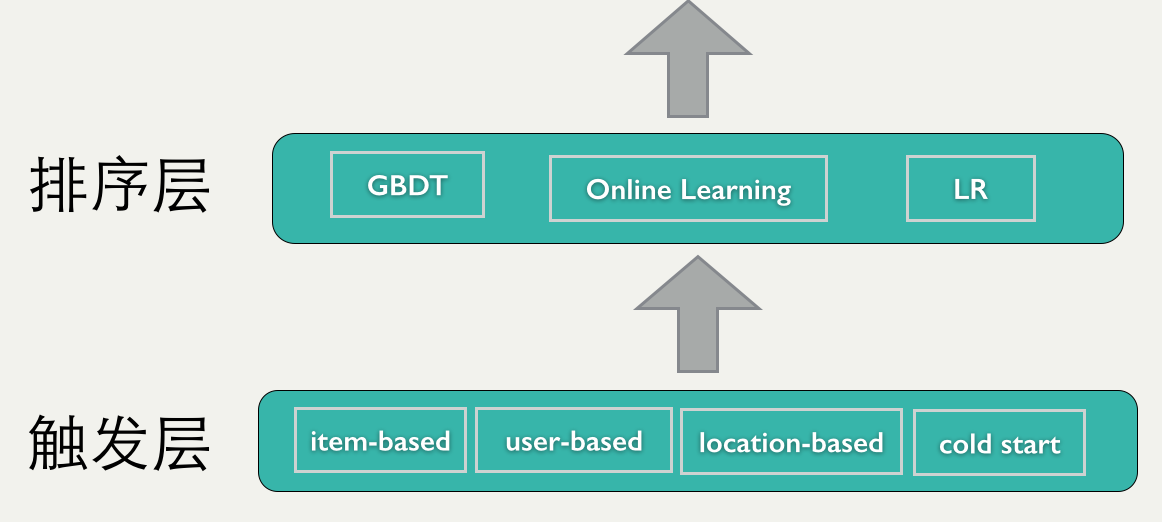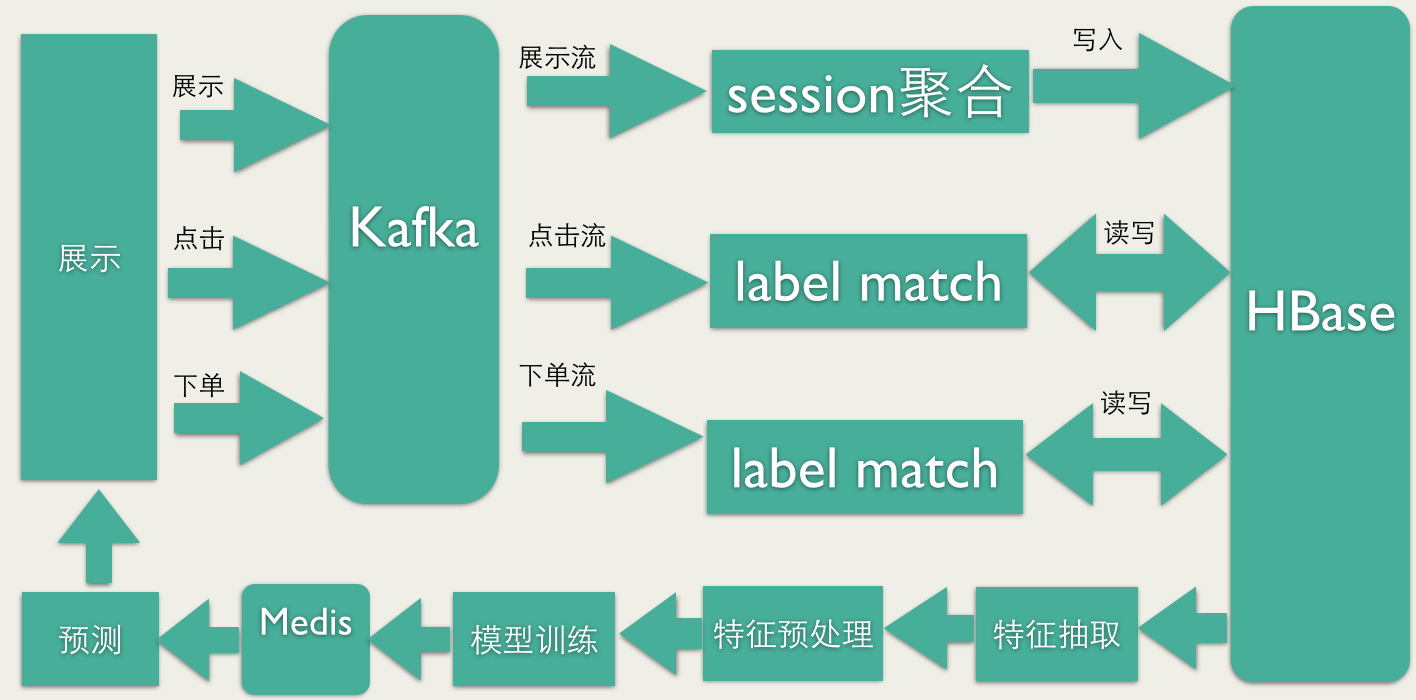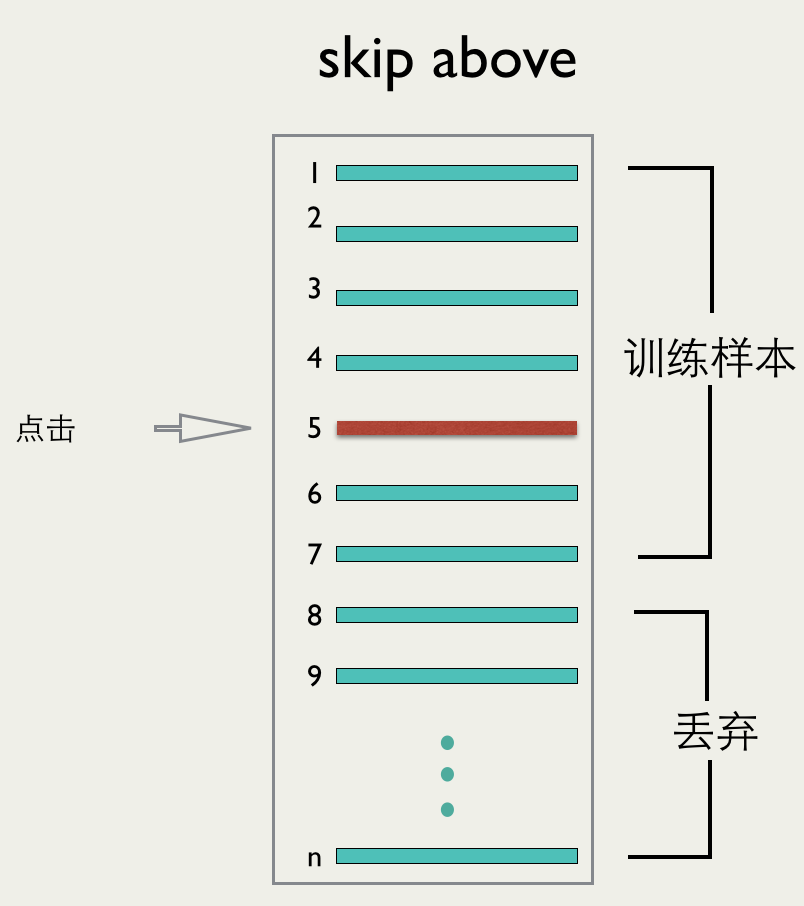FTRL0.8432200W
BPR0.84411500W

BPR的效果略好，但是我们线上选用了FTRL模型，主要原因是FTRL能够产生稀疏化的效果，训练出的模型会比较小。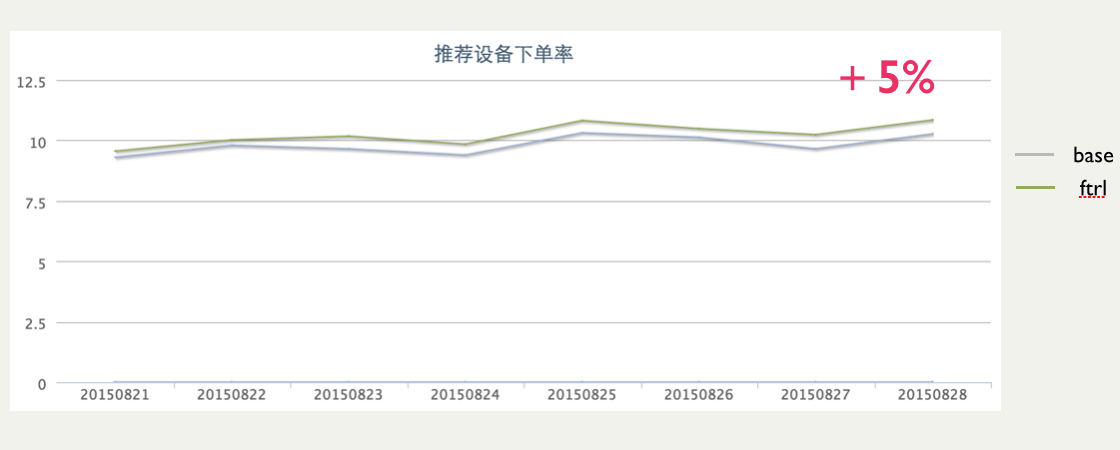## 参考资料

•  McMahan H B, Holt G, Sculley D, et al. Ad Click Prediction: a View from the Trenches. Proceedings of the 19th ACM SIGKDD International Conference on Knowledge Discovery and Data Mining (KDD). 2013.
•  Graepel T, Candela J Q, Borchert T,et al. Web-Scale Bayesian Click-Through Rate Prediction for Sponsored Search Advertising in Microsoft’s Bing Search Engine. Proceedings of the 27th International Conference on Machine Learning ICML. 2010.
•  Murphy K P. Machine Learning: A Probabilistic Perspective. The MIT Press. 2012.